Numbers: Getting to grips with division

This free course is available to start right now. Review the full course description and key learning outcomes and create an account and enrol if you want a free statement of participation.

Free course

# 11 Example of long division

The example of 25546 divided by 53 is suitable for long division. First write the calculation down on paper in the same way you did before.Figure 19

Look at the first digit of the number you are dividing into. This is 2, but 53 doesn’t go into 2. So you take the next digit as well, which makes it 25, but 53 doesn’t go into 25 either. So you take the next digit as well, which makes it 255.

Now you have to work out how many times 53 goes into 255. This step usually involves trying out multiples of 53. First make an estimate in your head. For example there are two 50s in a hundred, so there will be four 50s in 200. But 255 probably isn’t big enough for 53 multiplied by 5. So assume for the moment that the answer is 4 and work out what 53 multiplied by 4 is.

The result is 212 as shown below. This is less than 255, which it needs to be. It is also close enough to 255 to suggest that 53 multiplied by 5 would be bigger than 255, so 4 is probably right. Click on each step below in turn to see how to continue with long division.

Active content not displayed. This content requires JavaScript to be enabled.
Interactive feature not available in single page view (see it in standard view).

## Activity 4

Click on ‘Reveal discussion’ to see all the individual steps at once.

### Discussion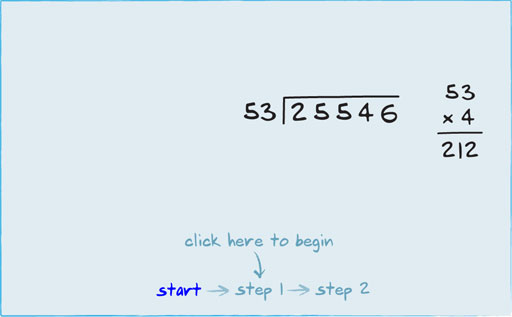Figure 20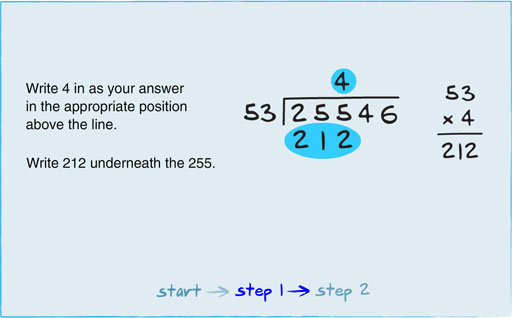Figure 21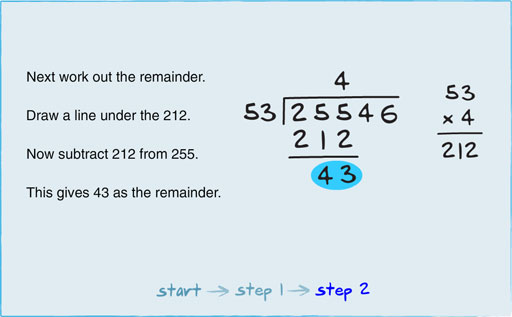Figure 22

At this point you can check whether your answer for this column is correct or not. You can’t have a remainder that is bigger than the number you are dividing by. So if the result of your subtraction is smaller than the number you are dividing by, then your answer for this column is correct. If it isn’t, then you need to multiply 53 again. In this case, the result of the subtraction is 43. This is smaller than 53, which is the number you are dividing by, so the answer of 4 is correct.

Next you need to carry the remainder and put it in front of the next digit, turning the 4 into 434. But if you have a big number to carry (and in divisions with big numbers the remainder could be several digits long), trying to write it into the small space between the 5 and the 4 is difficult. So instead, write the 4 after the remainder of 43, as shown below, to make it 434.

Now work out how many times 53 goes into 434. Do an estimate in your head then multiply it out to see if it is close enough to the total for any remainder to be less than the number you are dividing by. Click on each step below in turn to see how the long division is completed.

Active content not displayed. This content requires JavaScript to be enabled.
Interactive feature not available in single page view (see it in standard view).

## Activity 5

Click on ‘Reveal discussion’ to see all the individual steps at once.

### Discussion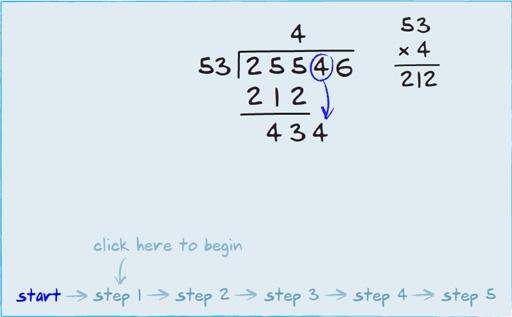Figure 23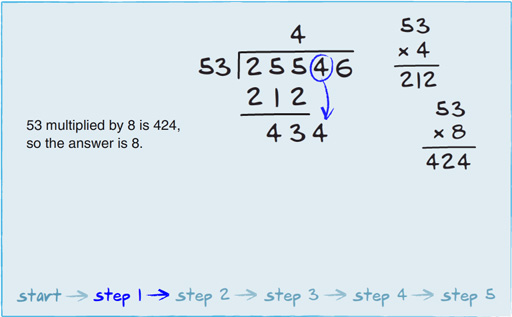Figure 24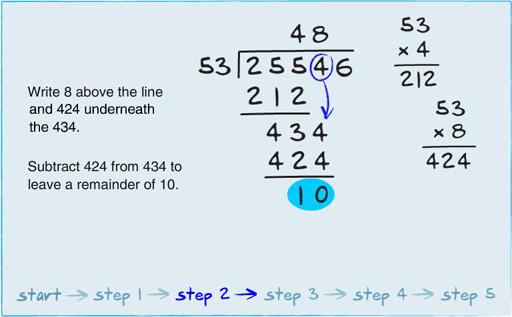Figure 25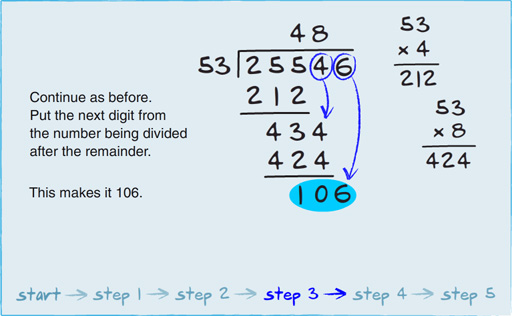Figure 26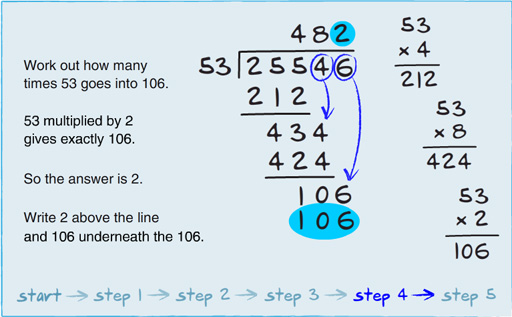Figure 27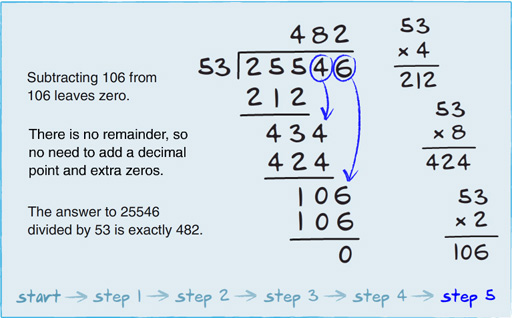Figure 28

So far, all the division calculations I’ve considered have involved one whole number divided by another whole number. What do you do if you want to do a division that involves decimal numbers? This is what the next section will look at.

Div_1

### Take your learning further

Making the decision to study can be a big step, which is why you'll want a trusted University. The Open University has 50 years’ experience delivering flexible learning and 170,000 students are studying with us right now. Take a look at all Open University courses.

If you are new to university level study, find out more about the types of qualifications we offer, including our entry level Access courses and Certificates.

Not ready for University study then browse over 900 free courses on OpenLearn and sign up to our newsletter to hear about new free courses as they are released.

Every year, thousands of students decide to study with The Open University. With over 120 qualifications, we’ve got the right course for you.

Request an Open University prospectus